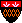Elements of ML Programming, 2nd Edition (ML97)

## Errata

p. 11, l. 6: 1*4096 + 2*256 + 3*16 + 4 = 4660 (Thanks to Steve Arons).

p. 25, Fig. 2.3: Header round should be round(x) (Thanks to Sing Lo).

p. 25, Fig. 2.3: Apparently in SML/NJ Version 110, rounding of half integers is upward in magnitude. Thus, round(~3.5) is now ~4 (Thanks to Cong-cong Xing). p. 25, last line: val it = #"a" : char (Thanks to John M. Linebarger).

p. 54, last line: ``than'' -> ``that'' (Thanks to Suan Lue).

p. 64, Ex. 3.2.1(f): Because there are complications due to the fact that real is not an equality type and the function null is not introduced until p. 319, the stipulation ``list of reals,'' is changed to ``list of strings'' (Thanks to Dirk Ullrich).

p. 83, Exercise 3.4.4: This exercise should refer to Exercise 3.2.1(f), not (e) (Thanks to David J. Meppelink).

p. 66: Function reverse needs a semicolon at the end (Thanks to Simone Scalabrin).

p. 75, l. 4: Should refer to Exercise 3.2.1(e) Also, l. 5 should refer to Exercise 3.2.1(f). (Thanks to Tom Maynard).

p. 75, l. -10: The pattern should be [((x,y),zs)] (Thanks to Cong Xing). Also, the correction needed a correction, which was provided by Brian Ammon. p. 76, Exercise 3.3.13: Should refer in the first line to Exercise 3.3.11 (Thanks to Nick Russo).

p. 94ff, Section 3.6.5: I received an email from Yekaterina Karatsuba. She is the daughter of Anatoly Karatsuba, one of the authors of the paper by Karatsuba and Ofman, from which the main idea of this section was taken. She points out that while both had their names on the paper, the result in question about a fast way to perform multiplication was attributed in the paper to her father alone. Thus, the idea should have been referred to simply as Karatsuba's algorithm.

p. 97, l. 14: TW should be TV (Thanks to Dario Rudoni).

p. 97, l. 19: TV+UW should be TW+UV (Thanks to David Schonberger).

p. 103, l. 8: Semicolon after "hello world" (Thanks to Sing Lo).

p. 109, l. -13: input should be inputN (Thanks to Dario Rudoni).

p. 110, Fig. 4.4 caption: ``and turning it into a list of 1-character strings'' (Thanks to Shengqing Zhou).

p. 116, Fig. 4.8: The 22nd is missing. Put it in the obvious place and move the 23rd through the 30th one day later (Thanks to Peter Amstutz).

p. 117, l. 12, 14: The arguments to openOut and openAppend are of the form "<file name>" (Thanks to David Meppelink).

p. 120, Exercise 4.3.3: This exercise is a duplicate of Exercise 4.1.3 and should be ignored (Thanks to Peter Amstutz).

p. 123, box, l. 5: The single quotes should be double quotes (Thanks to John D. Evans).

p. 130, l. -8: input1(file) should be input1(infile) (Thanks to David Schonberger).

p. 135, l. -3: ``Expressions'' -> ``Exceptions'' (Thanks to James Gary).

p. 140, last line of Fig. 5.5: ``nonconstructor'' -> ``non-constructor'' and ``OutofRange'' -> ``OutOfRange'' (Thanks to Shengqing Zhou).

p. 141, l. 7 of text: ``Fig. 5.3'' -> ``Fig. 5.5'' (Thanks to Shengqing Zhou).

p. 146, l. -10 to -8: Versions of SML after 109.30, on which this book is based, also allow lists of nonexpansive expressions to be nonexpansive (Thanks to Edgar Lederer).

p. 149, item (6): ceiling should be ceil and truncate should be trunc (Thanks to Shengqing Zhou).

p. 153, Fig. 5.10, l. (5): A semicolon is needed at the end (Thanks to David Schonberger).

p. 160, middle: semicolon missing from the end of function simpleMap (Thanks to David Schonberger). Also, the response should refer to function simpleMap (Thanks to Simone Scalabrin).

p. 164, l. 16: val missing from the response to function variance (Thanks to Simone Scalbrin).

p. 165, l. 4: Question-mark needed at end of sentence ``...Fig. 5.16?'' (Thanks to Simone Scalabrin).

p. 180, ML response in Fig. 5.23: the right parenthesis belongs after the second 'b, not the first (Thanks to Shengqing Zhou).

p. 181: fold should be foldr on lines 18 and -9 (Thanks to Shengqing Zhou).

p. 195, l. 5: This test won't work, because reals are not an equality type in ML97. The best fix is just to make everything in Example 6.1 integer, i.e., type signal = int list, val v = [1,2] : signal, etc. (Thanks to Aaron Leung).

p. 195, l. -14: Right parenthesis needed after "5.6.3" (Thanks to Omri Traub).

p. 197, l. 9: ``buld'' -> ``build'' (Thanks to Kok-Leung Chin).

p. 198, l. 3: ``letter in an identifiers'' -> ``letter of an identifier'' (Thanks to Suan Lue).

p. 205, response to line (4): Enode of 'a * 'a oddTree * 'a oddTree (Thanks to John D. Evans).

p. 220, l. -7: ``line (11)'' -> ``line (9)'' (Thanks to Shengqing Zhou).

p. 221, l. 14: val sum = fn: int btree -> int (Thanks to John D. Evans).

p. 256, l. -4: ``Example 7.7'' should be ``Fig. 7.7.''

p. 286, l. 14: ``is'' -> ``in'' (Thanks to Joohee Jeong).

p. 328, Exercise 9.3.1, l. 1: ``compare'' -> ``and compare.''

p. 378, first entry: ``Coersion'' -> ``Coercion'' (Thanks to Kim Bruce).

#### Corrections to Solutions

Thanks also to Maria Garcia de la Banda, Suchindra Rengan Omri Traub, and Cong Yan for corrections to the published solutions.